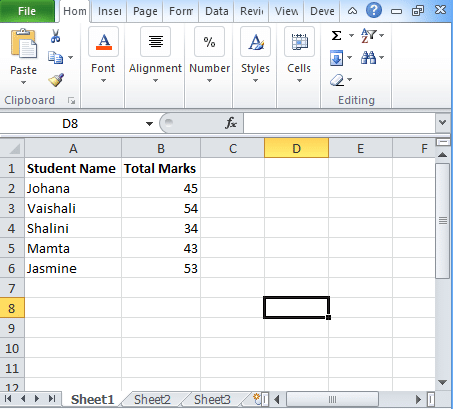To advertise with us contact on Whatsapp: +923041280395 For guest post email at: [email protected]

# Excel If Statement: Step by Step process to useWhen you want to perform any decision-making task then you always thought of If statement. If the statement is used to make the decision on the basis of certain criteria, it evaluates an expression and gives you an outcome. In the post, you will be going to see how to use Excel If statement.

## Excel If statement

The Excel If statement is used to define any function to check whether the certain condition met or not. On the basis of this, it will return an outcome.

### Syntax

=IF (Logic_Test, Value_if_True, Value_If_False)

Where Logic_Test refers to the expression that you want to evaluate.

Value_If_True gives you the outcome of the statement if the logical condition is true.

Value_If_False gives you the outcome of the statement if the test is false.

### NOTE:

At a single point in time, the Excel If the statement returns only one value.

You can use Nesting if statement but excel allows till 64 levels.

If you use If statement wrongly, then it shows an error “#Name?”.

## How to use Excel If Statement?

Example 1:

• To make you understand Excel If statement, we are taking data set of students shown above.
• In the table, there are two columns, one for the student name and the other one is for marks.
• Now we will calculate the result of the student whether he or she is passed or not on the basis of his total marks.
• While applying IF statement, a student whose score is more than 50 marks, he or she has passed the exam.
• Candidates whose score is less than 50, he or she failed the exam.
• The formula is =IF(B2<=50,”Fail”,”Pass”)

Example 2:

• Here we will take an example of the salaried employee. Calculate the bonus of the employees.
• To calculate the bonus, you have to follow criteria to give the bonus to the employees.
• According to the criteria, if the salary of the employee is greater than or equal to \$7000 then the bonus will be given 10% of the salary, else 5% bonus will be given.
• For this, we will take the formula =IF(B2>=\$7000, B2*10%,B2*5%).

This is how you will use Excel If Statement on numbers. In fact, you can also perform excel if statement multiple conditions range on different numeric values.

## Excel Nested If statement

People use this excel nested if statement to test different conditions. Check the syntax of excel nested if here:

If(condition1, result1, IF(condition2, result2, IF(condition3, result3, result4)))

In the nested if, you will test condition1, if TRUE, then returns result1, if false then it goes to test condition2. If True, then it returns result2, if false then test condition3. If true then returns result3, if false, then it returns result 4.

### If statement using logical operators

You can also use the Nested IF along with logical operators that are OR, AND to evaluate sets in different conditions. You can use Excel If statement with logical operators (AND, OR) to perform excel logical test and to analyze the complex logic behind this. If you use AND function, then it will result “TRUE” if all the condition met whereas OR function results “TRUE” if anyone condition met.